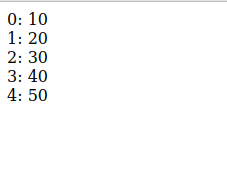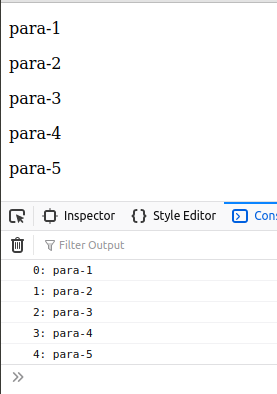Open in App
Not now

# What is the use of .each() function in jQuery ?

• Last Updated : 01 Jul, 2021

The each() function in jQuery iterate through both objects and arrays. Arrays that have length property are traversed from the index 0 to length-1 and whereas arrays like objects are traversed via their properties names.

Syntax:

```\$.each('array or object', function(index, value){
})```

In this .each() function, an array or an object is given as the first argument and a callback function. This callback function optionally takes two parameters that are index and value. Therefore, we have to pass a callback function to each() method.

Example 1:

## HTML

 ```<``html` `lang``=``"en"``>`` ` `<``head``>``    ````    ``<``script` `src``=``"https://code.jquery.com/jquery-git.js"``>``    `````` ` `<``body``>``  ``<``script``>``    ``let arr = [10, 20, 30, 40, 50];``    ``\$.each(arr, function (index, value) {``        ``document.write(index + ": " + value + "<``br``>");``    ``});``  `````` ` ``

Output:\$(selector).each(): We can also break the loop early by returning false from the callback function. It is the same as above each() function, but it iterates over the DOM elements of the JQuery object and can execute a function for every element.

Syntax:

```\$('selector').each(function(index, value){
})```

It only accepts a callback function that executes for each selected element.

Example:

## HTML

 ```<``html` `lang``=``"en"``>`` ` `<``head``>``    ````    ``<``script` `src``=``"https://code.jquery.com/jquery-git.js"``>``    `````` ` `<``body``>``    ``<``p``>para-1``    ``<``p``>para-2``    ``<``p``>para-3``    ``<``p``>para-4``    ``<``p``>para-5``    ``<``script``>``        ``\$("p").each(function (index) {``            ``console.log(index ``                ``+ ": " + \$(this).text());``        ``});``    `````` ` ``

Output:My Personal Notes arrow_drop_up